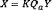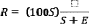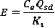# Title 40

## SECTION 60.5406

### 60.5406 What test methods and procedures must I use for my sweetening units affected facilities at onshore natural gas processing plants

§ 60.5406 What test methods and procedures must I use for my sweetening units affected facilities at onshore natural gas processing plants?

(a) In conducting the performance tests required in § 60.8, you must use the test methods in appendix A of this part or other methods and procedures as specified in this section, except as provided in paragraph § 60.8(b).

(b) During a performance test required by § 60.8, you must determine the minimum required reduction efficiencies (Z) of SO2 emissions as required in § 60.5405(a) and (b) as follows:

(1) The average sulfur feed rate (X) must be computed as follows:Where: X = average sulfur feed rate, Mg/D (LT/D). Qa = average volumetric flow rate of acid gas from sweetening unit, dscm/day (dscf/day). Y = average H2S concentration in acid gas feed from sweetening unit, percent by volume, expressed as a decimal. K = (32 kg S/kg-mole)/((24.04 dscm/kg-mole)(1000 kg S/Mg)). = 1.331 × 10−3Mg/dscm, for metric units. = (32 lb S/lb-mole)/((385.36 dscf/lb-mole)(2240 lb S/long ton)). = 3.707 × 10−5 long ton/dscf, for English units.

(2) You must use the continuous readings from the process flowmeter to determine the average volumetric flow rate (Qa) in dscm/day (dscf/day) of the acid gas from the sweetening unit for each run.

(3) You must use the Tutwiler procedure in § 60.5408 or a chromatographic procedure following ASTM E260-96 (incorporated by reference as specified in § 60.17) to determine the H2S concentration in the acid gas feed from the sweetening unit (Y). At least one sample per hour (at equally spaced intervals) must be taken during each 4-hour run. The arithmetic mean of all samples must be the average H2S concentration (Y) on a dry basis for the run. By multiplying the result from the Tutwiler procedure by 1.62 × 10−3, the units gr/100 scf are converted to volume percent.

(4) Using the information from paragraphs (b)(1) and (b)(3) of this section, Tables 1 and 2 of this subpart must be used to determine the required initial (Zi) and continuous (Zc) reduction efficiencies of SO2 emissions.

(c) You must determine compliance with the SO2 standards in § 60.5405(a) or (b) as follows:

(1) You must compute the emission reduction efficiency (R) achieved by the sulfur recovery technology for each run using the following equation:(2) You must use the level indicators or manual soundings to measure the liquid sulfur accumulation rate in the product storage vessels. You must use readings taken at the beginning and end of each run, the tank geometry, sulfur density at the storage temperature, and sample duration to determine the sulfur production rate (S) in kg/hr (lb/hr) for each run.

(3) You must compute the emission rate of sulfur for each run as follows:Where: E = emission rate of sulfur per run, kg/hr. Ce = concentration of sulfur equivalent (SO2+ reduced sulfur), g/dscm (lb/dscf). Qsd = volumetric flow rate of effluent gas, dscm/hr (dscf/hr). K1 = conversion factor, 1000 g/kg (7000 gr/lb).

(4) The concentration (Ce) of sulfur equivalent must be the sum of the SO2 and TRS concentrations, after being converted to sulfur equivalents. For each run and each of the test methods specified in this paragraph (c) of this section, you must use a sampling time of at least 4 hours. You must use Method 1 of appendix A to part 60 of this chapter to select the sampling site. The sampling point in the duct must be at the centroid of the cross-section if the area is less than 5 m 2 (54 ft 2) or at a point no closer to the walls than 1 m (39 in) if the cross-sectional area is 5 m 2 or more, and the centroid is more than 1 m (39 in.) from the wall.

(i) You must use Method 6 of appendix A to part 60 of this chapter to determine the SO2 concentration. You must take eight samples of 20 minutes each at 30-minute intervals. The arithmetic average must be the concentration for the run. The concentration must be multiplied by 0.5 × 10−3 to convert the results to sulfur equivalent.

(ii) You must use Method 15 of appendix A to part 60 of this chapter to determine the TRS concentration from reduction-type devices or where the oxygen content of the effluent gas is less than 1.0 percent by volume. The sampling rate must be at least 3 liters/min (0.1 ft 3/min) to insure minimum residence time in the sample line. You must take sixteen samples at 15-minute intervals. The arithmetic average of all the samples must be the concentration for the run. The concentration in ppm reduced sulfur as sulfur must be multiplied by 1.333 × 10−3 to convert the results to sulfur equivalent.

(iii) You must use Method 16A or Method 15 of appendix A to part 60 of this chapter or ANSI/ASME PTC 19.10-1981, Part 10 (manual portion only) (incorporated by reference as specified in § 60.17) to determine the reduced sulfur concentration from oxidation-type devices or where the oxygen content of the effluent gas is greater than 1.0 percent by volume. You must take eight samples of 20 minutes each at 30-minute intervals. The arithmetic average must be the concentration for the run. The concentration in ppm reduced sulfur as sulfur must be multiplied by 1.333 × 10−3 to convert the results to sulfur equivalent.

(iv) You must use Method 2 of appendix A to part 60 of this chapter to determine the volumetric flow rate of the effluent gas. A velocity traverse must be conducted at the beginning and end of each run. The arithmetic average of the two measurements must be used to calculate the volumetric flow rate (Qsd) for the run. For the determination of the effluent gas molecular weight, a single integrated sample over the 4-hour period may be taken and analyzed or grab samples at 1-hour intervals may be taken, analyzed, and averaged. For the moisture content, you must take two samples of at least 0.10 dscm (3.5 dscf) and 10 minutes at the beginning of the 4-hour run and near the end of the time period. The arithmetic average of the two runs must be the moisture content for the run.